• 目前我想标定加速度传感器的幅频特性曲线以及相位差 然后我用振动台标定加速度传感器，加速度传感器放在台面上，同时用激光位移计记录位移。输入为激光位移传感器的二次微分，输出为加速度传感器。 然后目前我想得到...matlab 有问必答 测试工具
• 幅频特性和相频特性 G(jω)称为频率特性，A(ω)是输出信号的幅值与输入信号幅值之比，称为幅频特性。 Φ(ω)是输出信号的相角与输入信号的相角之差，称为相频特性。 相移角度随频率变化的特性叫相频特性。 由于放大...
幅频特性和相频特性
G(jω)称为频率特性，A(ω)是输出信号的幅值与输入信号幅值之比，称为幅频特性。 Φ(ω)是输出信号的相角与输入信号的相角之差，称为相频特性。 相移角度随频率变化的特性叫相频特性。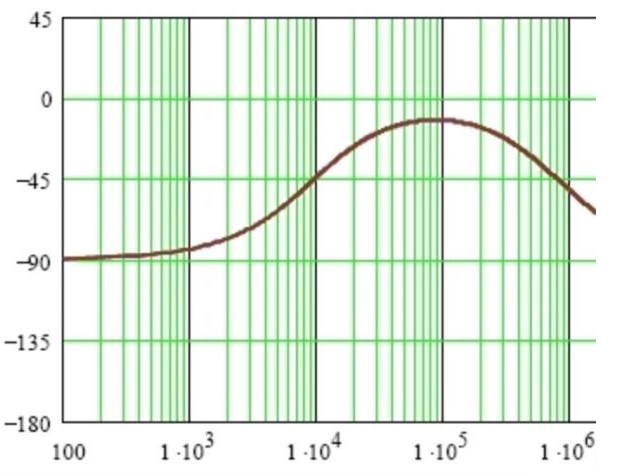由于放大电路中电抗元件的存在，放大电路对不同频率分量的信号放大能力是不相同的，而且不同频率分量的信号通过放大电路后还会产生不同的相移。因此，衡量放大电路放大能力的放大倍数也就成为频率的函数。 放大电路的电压放大倍数与频率的关系称为幅频特性,输出信号与输入信号的相位差与频率之间的关系称为相频特性。两者统称频率特性。
展开全文• 基于STM32F407的幅频特性和相频特性测试仪，内含设计主要源码。
• matlab画频率响应曲线的函数为： [h,w] = freqz(b,a,n) b,a：传递函数系数 h：频率响应 w：角频率，0~π 更多参数解释参考官方链接...
matlab画频率响应曲线的函数为：
[h,w] = freqz(b,a,n)
b,a：传递函数系数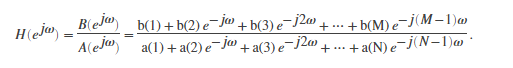h：频率响应
w：角频率，0~π
更多参数解释参考官方链接https://ww2.mathworks.cn/help/signal/ref/freqz.html?requesteddomain=true
用freqz画频率响应曲线的一个例子：绘制如下系统的频响曲线： H(z)=(1-0.5z^-1)
B=[1 -0.5];
A =;
[H,w]=freqz(B,A);
Hf=abs(H);  %取幅度值实部
Hx=angle(H);  %取相位值对应相位角
clf
figure(1)
plot(w,20*log10(Hf))  %幅值变换为分贝单位
title('离散系统幅频特性曲线')
figure(2)
plot(w,Hx)
title('离散系统相频特性曲线')
幅频特性曲线：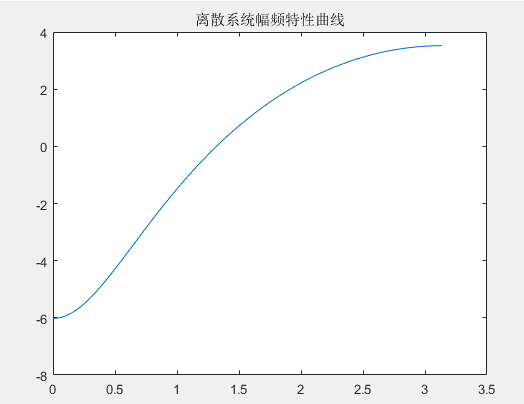此时幅频特性曲线的横坐标为数字角频率w。有时候我们想把横坐标转换为频率f（以赫兹hz为单位）：
首先要弄懂各个频率之间的关系：
模拟频率f：每秒经历多少个周期，单位Hz，即1/s；
各频率之间的关系：
Ω=2*π*f；
w =Ω*T；
因为T=1/fs（fs为采样率）
所以w =Ω*T=2*π*f/fs。
因此将数字角频率w转换为模拟频率f的公式为：
f=w*fs/(2*π)
因此，将横坐标转换为以赫兹为单位的代码如下（假设采样率为1000hz）：
B=[1 -0.5];
A =;
[H,w]=freqz(B,A);
Hf=abs(H);  %取幅度值实部
Hx=angle(H);  %取相位值对应相位角
clf
figure(1)
plot(w*fs/(2*pi),20*log10(Hf))  %幅值变换为分贝单位
title('离散系统幅频特性曲线')
figure(2)
plot(w*fs/(2*pi),Hx)
title('离散系统相频特性曲线')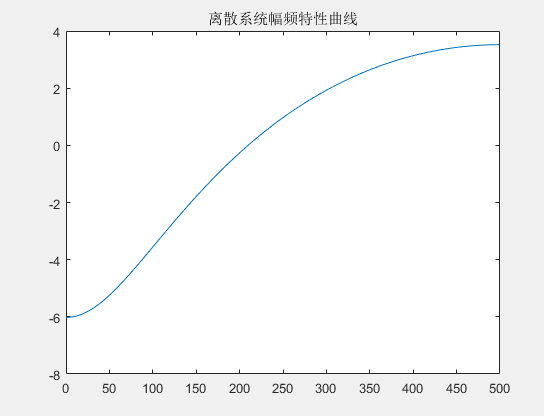此时幅频特性曲线的横坐标为频率f，单位为hz
ps：
当幅频特性曲线的横坐标为数字角频率w时，其最大值为π，这是因为当采样率为fs时，根据香农定理，能够采样的信号的最高频率为fs/2，fs/2频率对应的数字角频率w即为π。因此通常我们只关心0-π的幅频响应。
从上面最后一张图可以看到，当横坐标转换为频率后，横坐标最大值为fs/2，即500hz。
展开全文• 第28章 FFTIFFT的Matlab实现（幅频响应和相频响应） 本章主要讲解fft，ifftfftshift在matlab上的实现。 目录 第28章 FFTIFFT的Matlab实现（幅频响应和相频响应） 28.1 初学者重要提示 28.2 Matlab的FFT...
完整版教程下载地址：http://www.armbbs.cn/forum.php?mod=viewthread&tid=94547
第28章       FFT和IFFT的Matlab实现（幅频响应和相频响应）
本章主要讲解fft，ifft和fftshift在matlab上的实现。
目录
第28章       FFT和IFFT的Matlab实现（幅频响应和相频响应）
28.1 初学者重要提示
28.2  Matlab的FFT函数
28.2.1        函数语法
28.2.2        函数定义
28.2.3        函数描述
28.2.4        FFT实例一：幅频响应
28.2.5        FFT实例二：相频响应（重要）
28.3 Matlab的IFFT函数
28.3.1        函数语法
28.3.2        函数描述
28.3.3        IFFT实例
28.4 Matlab的FFTSHIFT函数
28.5 总结

28.1 初学者重要提示
求解FFT相频时的修正比较重要，本章做了一个简易修正方法。重点看28.2.5小节。
28.2  Matlab的FFT函数
28.2.1        函数语法
Y = fft(x)
Y = fft(X,n)
Y = fft(X,n,dim)
28.2.2        函数定义
Y = fft(x) 和 y = ifft(X)分别用于实现正变换和逆变换，公式描述如下：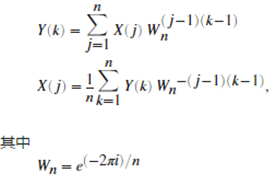28.2.3        函数描述
Y = fft(X)
用快速傅里叶变换 (FFT) 算法计算 X 的离散傅里叶变换 (DFT)。
如果 X 是向量，则 fft(X) 返回该向量的傅里叶变换。  如果 X 是矩阵，则 fft(X) 将 X 的各列视为向量，并返回每列的傅里叶变换。  如果 X 是一个多维数组，则 fft(X) 将尺寸大小不等于 1 的第一个数组维度的值视为向量，并返回每个向量的傅里叶变换。
注意这里第一个尺寸不为1是指一个矩阵的第一个尺寸不为1的维。
比如一个矩阵是2*1，那么第一个尺寸不为1的维就是行（尺寸为2）。
X是 1*2*3表示第一个尺寸不为1的维就是列（尺寸为2）。
X为维数5*6*2的话，第一个尺寸不为1的维就是行（尺寸为5）。

Y = fft(X, n)
返回 n 点 DFT。如果未指定任何值，则 Y 的大小与 X 相同。
如果 X 是向量且 X 的长度小于 n，则为 X 补上尾零以达到长度 n。  如果 X 是向量且 X 的长度大于 n，则对 X 进行截断以达到长度 n。  如果 X 是矩阵，则每列的处理与在向量情况下相同。  如果 X 为多维数组，则大小不等于 1 的第一个数组维度的处理与在向量情况下相同。

Y = fft(X, n, dim)
返回沿维度 dim 的傅里叶变换。例如，如果 X 是矩阵，则 fft(X,n,2) 返回每行的 n 点傅里叶变换。
28.2.4        FFT实例一：幅频响应
傅里叶变换的一个常见用途就是查找埋藏在噪声信号中的实际信号的频率成分。下面我们考虑一个这样的例子：
采样率是1000Hz ，信号由如下三个波形组成。
（1）50Hz的正弦波、振幅0,7。
（2）70Hz正弦波、振幅1。
（3）均值为0的随机噪声。
实际运行代码如下：
Fs = 1000;             %采样率
T = 1/Fs;              %采样时间单位
L = 1000;              %信号长度
t = (0:L-1)*T;         %时间序列

x = 0.7*sin(2*pi*50*t) + sin(2*pi*120*t);  %原始信号
y = x + 2*randn(size(t));                  %原始信号叠加了噪声后
plot(Fs*t(1:50),y(1:50));                  %绘制波形
title('原始信号+零均值随机噪声 ');
xlabel('时间单位:ms');
运行Matlab后，显示波形如下：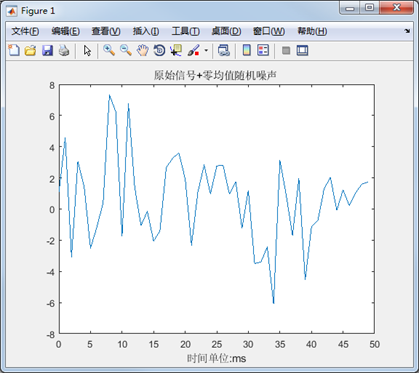通过上面的截图，我们是很难发现波形中的频率成分，下面我们通过FFT变换，从频域观察就很方便了，Matlab运行代码如下：
Fs = 1000;           %采样率
T = 1/Fs;            %采样时间单位
L = 1000;            %信号长度
t = (0:L-1)*T;       %时间序列

x = 0.7*sin(2*pi*50*t) + sin(2*pi*120*t);  %原始信号
y = x + 2*randn(size(t));                  %原始信号叠加了噪声后

NFFT = 2^nextpow2(L);            %求得最接近采样点的2^n，由于上面是1000点，那么最近的就是1024点。
Y=fft(y,NFFT)/L;                 % 进行FFT变换，除以总的采样点数，方便观察实际值。
f = Fs/2*linspace(0,1,NFFT/2+1); %频率轴，这里只显示Fs/2部分，另一半是对称的。

plot(f,2*abs(Y(1:NFFT/2+1)))     %绘制波形
title('幅频相应');
xlabel('频率');
ylabel('幅度');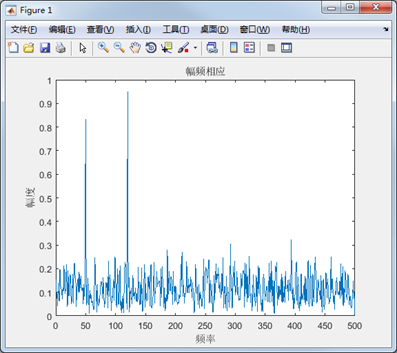从上面的幅频相应，我们可以看出，两个正弦波的频谱并不是准确的0.7和1，而是比较接近，这个就是我们在上节教程中所示的频谱泄露以及噪声的干扰。
28.2.5        FFT实例二：相频响应（重要）
这里我们以采样两个余弦波组成的信号为例进行说明，并求出其幅频和相频响应。
（1）50Hz的余弦波，初始相位60°，振幅1.5。
（2）90Hz的余弦波、初始相位60°，振幅1。
（3）采样率256Hz，采集256个点。

Matlab上面运行的代码如下：
Fs = 256;              % 采样率
N  = 256;             % 采样点数
n  = 0:N-1;            % 采样序列
t  = 0:1/Fs:1-1/Fs;     % 时间序列
f = n * Fs / N;          %真实的频率

x = 1.5*cos(2*pi*50*t+pi/3)+ cos(2*pi*90*t+pi/3) ;  %原始信号
y = fft(x, N);    %对原始信号做FFT变换
Mag = abs(y);    %求FFT转换结果的模值
subplot(2,1,1);
plot(f, Mag);       %绘制幅频相应曲线
title('幅频相应');
xlabel('频率/Hz');
ylabel('幅度');

subplot(2,1,2);
plot(f,  angle(y)*180/pi);   %绘制相频响应曲线，注意这将弧度转换成了角度
title('相频响应方式一');
xlabel('频率/Hz');
ylabel('相角');
运行后求出的幅频相应和相频响应结果如下：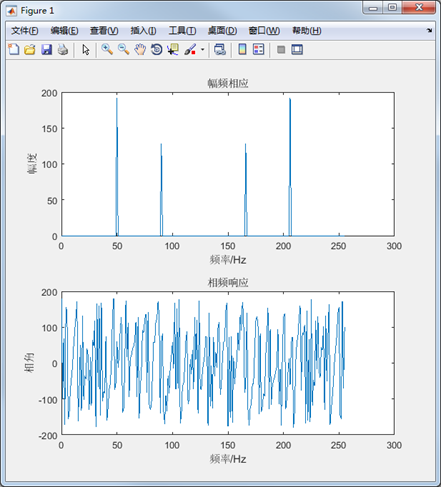求出的幅频响应没问题，而相频响应杂乱无章，造成这个问题的根本原因很多频段的幅值非常小，他们的相角可以不显示出来，这样就可以方便的查看相频响应了。基于此，修正后的代码如下：
Fs = 256;              % 采样率
N  = 256;             % 采样点数
n  = 0:N-1;            % 采样序列
t  = 0:1/Fs:1-1/Fs;     % 时间序列
f = n * Fs / N;          %真实的频率

x = 1.5*cos(2*pi*50*t+pi/3)+ cos(2*pi*90*t+pi/3) ;  %原始信号
y = fft(x, N);    %对原始信号做FFT变换
Mag = abs(y);    %求FFT转换结果的模值
subplot(3,1,1);
plot(f, Mag);       %绘制幅频相应曲线
title('幅频相应');
xlabel('频率/Hz');
ylabel('幅度');

subplot(3,1,2);
plot(f,  angle(y)*180/pi);   %绘制相频响应曲线，注意这将弧度转换成了角度
title('相频响应方式一');
xlabel('频率/Hz');
ylabel('相角');

subplot(3,1,3);
plot(f,  angle(y)*180/pi.*(Mag>=100)); %绘制相频响应曲线，注意这将弧度转换成了角度
title('相频响应方式二');
xlabel('频率/Hz');
ylabel('相角');
上面代码中Mag>=100是关键，仅展示FFT后幅值大于100的相角。下面再来看Matlab的效果：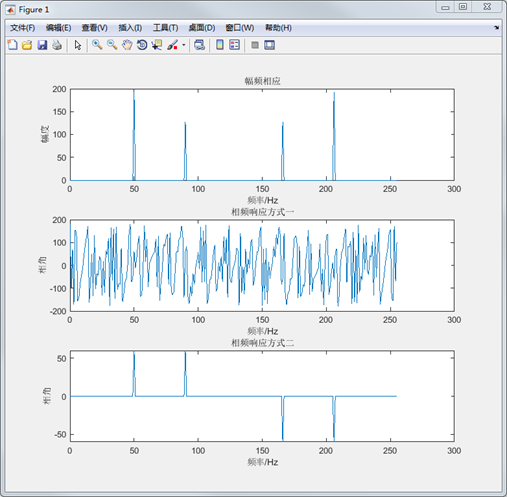可以看到已经完全没问题了，求出了频率50Hz的余弦初相为60°左右，频率90Hz的余弦初相也是60°。
28.3 Matlab的IFFT函数
28.3.1        函数语法
y = ifft(X)
y = ifft(X,n)
y = ifft(X,[],dim)
y = ifft(X,n,dim)
y = ifft(..., 'symmetric')
y = ifft(..., 'nonsymmetric')
28.3.2        函数描述
y = ifft(X)
此函数用于返回向量X的离散傅立叶变换（DFT）逆变换结果，计算时使用快速傅里叶算法（Fast Fourier transform (FFT)）。
y = ifft(X,n)
此函数用于返回n点的IDFT。
y = ifft(X,[ ],dim)
y = ifft(X,n,dim)
上面两个函数用于实现指定维度的IFFT运算。
28.3.3        IFFT实例
下面我们对信号：0.7*sin(2*pi*50*t) + sin(2*pi*120*t)求FFT和IFFT，并绘制原始信号和转换后的信号。Matlab上运行的代码如下：
Fs = 1000;              %采样率
T = 1/Fs;               % 采样时间
L = 1024;               % 信号长度
t = (0:L-1)*T;          % 时间序列

y = 0.7*sin(2*pi*50*t) + sin(2*pi*120*t);  %50Hz正弦波和120Hz的正弦波的叠加

subplot(2,1,1);
plot(Fs*t(1:50), y(1:50));   %绘制原始信号
title('原始信号');

Y = fft(y, L);               %分别调用正变换和逆变换
Z = ifft(Y);
subplot(2,1,2);
plot(Fs*t(1:50), Z(1:50));   %绘制逆变换后的波形
title('FFT和IFFT转换后的信号');
运行后求出的结果如下：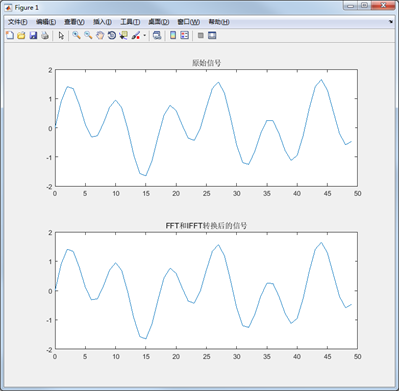通过上面的运行结果可以看出，转换后的波形与原始的波形基本是一样的。
28.4 Matlab的FFTSHIFT函数
fftshift的作用正是让正半轴部分和负半轴部分的图像分别关于各自的中心对称。因为直接用fft得出的数据与频率不是对应的，fftshift可以纠正过来
以下是Matlab的帮助文件中对fftshift的说明：
Y = fftshift(X) rearranges the outputs of fft, fft2, and fftn by moving the zero-frequency component to the center of the array. It is useful for visualizing a Fourier transform with the zero-frequency component in the middle of the spectrum. For vectors, fftshift(X) swaps the left and right halves of X。
下面我们在Matlab上面实现一个如下的代码来说明fftshift的使用：
Fs = 256;              % 采样率
N  = 256;              % 采样点数
n  = 0:N-1;            % 采样序列
t  = 0:1/Fs:1-1/Fs;    % 时间序列
f = (-N/2:N/2-1) * Fs / N;   %真实的频率

x = 1.5*sin(2*pi*50*t+pi/3) ;  %原始信号
y = fft(x, N);      %对原始信号做FFT变换
Mag = abs(y);    %求FFT转换结果的模值
subplot(2,1,1);
plot(f, Mag);       %绘制幅频相应曲线
title('fft幅频相应');
xlabel('频率/Hz');
ylabel('幅度');

z = fftshift(y);    %对FFT转换后的结果做偏移。
subplot(2,1,2);
plot(f, z);        %绘制幅频相应曲线
title('fftshift幅频相应');
xlabel('频率/Hz');
ylabel('幅度');
Matlab的运行结果如下：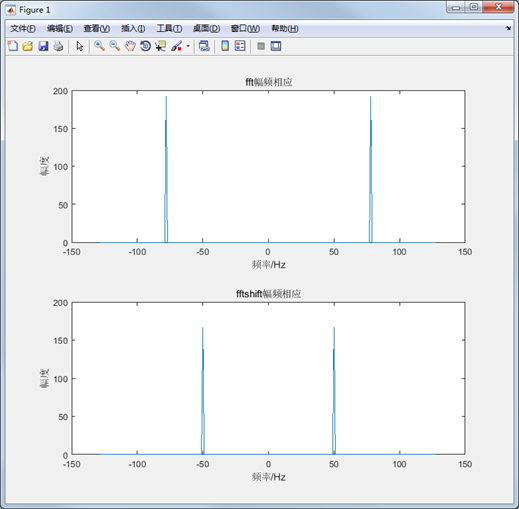通过上面的运行结果我们可以看到，经过fftshift的调节后，正弦波的中心频率正好对应在了相应的50Hz频率点。使用fftshift还有很多其它的好处，有兴趣的可以查找相关的资料进行了解。
28.5 总结
本章节主要讲解了fft，iff和fftshift的基本用法，如果要深入了解，一定要多练习，多查资料和翻阅相关书籍。

展开全文• 引言好多人学习数字信号处理学了很久都没有明白这两个概念的真实含义，或者说很多人在设计滤波器的时候，根本就没有考虑什么是幅频响应和相频响应。只是一昧地把滤波器设计出来然后把不要的频率滤除掉，这样是要吃大...

引言
好多人学习数字信号处理学了很久都没有明白这两个概念的真实含义，或者说很多人在设计滤波器的时候，根本就没有考虑什么是幅频响应和相频响应。只是一昧地把滤波器设计出来然后把不要的频率滤除掉，这样是要吃大亏的。鉴于此，我们来谈谈这两个概念，东西不难，只是大家伙儿没有用心去思考和理解罢了。还有一个因素就是老师没有跟大家讲明白，这个不用多说，现在的大学本科大家都懂是怎么回事。
首先我们要清楚一点就是幅频响应和相频响应针对的是一个系统，换句话说就是这两个概念是用来描述系统的状态和特性的，这是本文讨论的立足点和出发点。
幅频响应和相频响应的定义
幅频响应是探讨频率与其幅度的关系，一个信号通常包含很多频率，通过某电路时，不同频率所获得的增益(或衰减)是不同的，要探讨的就是要如何避免或者降低这些不一致所造成的影响。而相频响应，则是探讨不同频率通过某电路时所造成的相位偏移问题，所谓相位偏移其实就是时间延迟，我们根据 COSwt 可知相位是时间的函数，所以直接理解成时间延迟更加直观。
所以我们给系统一个正弦输入信号，系统输出响应随着正弦输入信号的角频率w而呈现不同的变化。其中输出幅度随着w变化规律为幅频特性，输出相角与输出相角的相位差与w的变化规律构成相频特性。
运放中的幅频特性和相频特性
在放大器中,放大倍数随频率变化的关系为：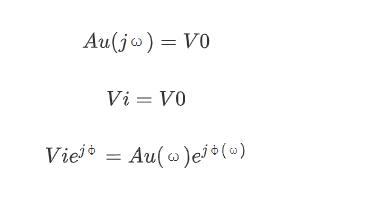式中 Au(w) 表示电压放大倍数的大小和频率之间的关系,称为幅频特性。幅频特性分为低通、高通、带通、带阻和全通。
输入信号与输出信号的相角差称为相频特性。相角差与频率的关系曲线称为相频特性曲线，正弦输入信号的响应也是正弦信号，频率与输入信号相同,只是经过系统会有一定的延迟。
物理意义
我们为什么要讨论这个话题？换句话说就是幅频响应和相频响应对于我们设计滤波器有什么用，也就是说其物理意义是什么？
滤波器的相频响应描述了在任意角频率为w的余弦(或正弦)信号激励下，滤波器没有非线性失真时的滤波器响应与激励之间的相位差。实际上就是描述(或正弦)信号激励下，滤波器没有非线性失真时的滤波器响应与激励之间的相位差。信号在滤波器中传输带来的延时情况，一般情况下，信号在动态电路中传输时出现延时，如果对所有频率信号产生的延时相等，则滤波器的相频特性曲线是一条直线，如下图所示。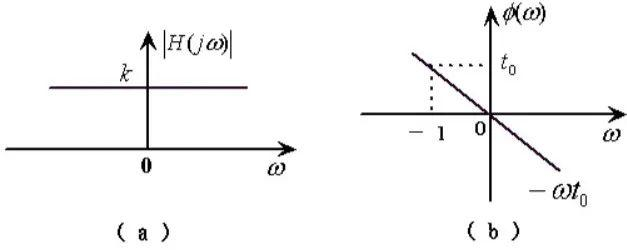那么还有一个问题就是，为什么要保持一条直线？这个就是我们要说明的真实问题，信号通过一个系统，如果各个频率成分的延时作用不一致，就会导致输入信号和输出信号不一致，导致严重的失真，那么这个对于我们的研究就没有意义。所以我们必须要保持所有的频率成分的延迟效果一直，也就是线性相位变化，这样的话，我们的输出和输入信号之间的差别就只有一个延迟效果，信号携带的信息成分并没有被破坏。
产生延迟效果的原因是因为信号经过系统被“处理了一下”，数字滤波器的话，计算也需要时间。我想在我们设计滤波器的时候这个问题必须考虑，不然设计出来的滤波器就没有用，也不敢用。而幅频响应表示的就是那些频率成分是这个系统允许通过的，能过的幅值较大，不能过的就抑制，幅值较小；所以一般滤波器都需要考虑这两个方面得到因素。

展开全文• 第28章 FFTIFFT的Matlab实现（幅频响应和相频响应） 本章主要讲解fft，ifftfftshift在matlab上的实现。 目录 第28章 FFTIFFT的Matlab实现（幅频响应和相频响应） 28.1 初学者重要提示 28.2 Matlab的FFT...FFT IFFT CMSIS DSP MATLAB
• 第28章 FFTIFFT的Matlab实现（幅频响应和相频响应） 本章主要讲解fft，ifftfftshift在matlab上的实现。 目录 第28章 FFTIFFT的Matlab实现（幅频响应和相频响应） 28.1 初学者重要提示 28.2 Matlab的FFT...
• Φ(ω)是输出信号的相角与输入信号的相角之差，称为相频特性（相移角度随频率变化的特性叫相频特性） 在‘信号与系统’理论里边，有一个重要的概念，叫做“系统的频率响应函数”，它的物理意义是：当系统的输入是一...
• 系统函数的幅频特性和相频特性分析参考与平台freqs(b,a,w)介绍例如输出 参考与平台 书籍《信号与系统》 matlab2016a freqs(b,a,w)介绍 对于有理分式，MATLAB 提供 freqs 函数处理方法。其调用格式为： H=freqs(b,a,w...
• 本程序使用matlab脚本分析闭环系统的频率特性，画出系统的幅频特性和相频特性曲线，即闭环伯德图。并直接得出该闭环系统的频率特性指标用来分析系统的稳定性。可以得到的指标有：谐振频率Wr、谐振峰值Mr、幅频带宽、...matlab
• 输入系统的参数得到系统的系统的输入输出、单位抽样响应、幅频响应特性曲线相频响应特性曲线、极一零图
• matlab仿真一阶低通滤波器幅频特性和相频特性.docx freqs模拟滤波器的频率响应语法：h=freqs(b,a,w)[h,w]=freqs(b,a)[h,w]=freqs(b,a,f)freqs(b,a)描述：freqs返回一个模拟滤波器的H(jw)的复频域响应(拉普拉斯格式)...
• 《matlab仿真一阶低通滤波器幅频特性和相频特性[计算机类]》由会员分享，可在线阅读，更多相关《matlab仿真一阶低通滤波器幅频特性和相频特性[计算机类](4页珍藏版)》请在人人文库网上搜索。1、freqs模拟滤波器的...keil mdk
• 已知y=x , x取值范围为〔0，2〕，如何用matlab 画幅频特性曲线和相频特性曲线matlab
• 不同阻尼比情况下幅频曲线（放大因子随频率比变化曲线）和相频曲线结构动力学
• 已知通带截止频率850Hz，阻带截止频率2000Hz，波纹3DB，衰减25DB，采样频率10kHz，求数字低通巴特沃斯滤波器阶数，归一化转折频率和幅频相频特性曲线。 clc ; wp = 850;ws=2000; wc = 5000; wp = wp / wc...
• 傅里叶变换求解相频幅频，实频虚频，曲线的matlab代码
• 1.起因 : 由于放大电路中电抗元件的存在,放大电路对不同频率分量的信号放大能力是不相同的(幅频特性提出点),而且不同频率分量的信号通过放大电路后还会产生不同的相移(相频特性提出点).因此衡量电路放大能力的放大...信号处理 经验分享
• 1. 控制系统幅频特性曲线的绘制步骤 改写系统传递函数 系统各典型环节幅频特性相乘（相频特性相加） 求特殊点 求特殊幅频和相频 绘制曲线 例子
• 信号幅频相频特性的画法(频率响应法)。频率响应法信号幅频相频特性的画法。信号与系统
• Excel中绘制幅频特性曲线的详细步骤设置，步骤
• 该系统基于扫频外差基本原理，以单片机和FPGA构成的最小系统为控制核心，可在任意指定频段内测量被测网络的幅频和相频特性并显示相应曲线。系统分DDS扫频信号源、被测网络...并在示波器上同时显示幅频曲线和相频曲线
• 截止频率为14Hz的一阶RC二阶RC滤波器幅频相频特性及阶跃响应Matlab
• 有源滤波器幅频特性曲线对数坐标纸，可以直接打印出来绘制有源滤波器特性曲线
• 结合伯德图奈奎斯特图，介绍增益裕度、相角裕度带宽等的概念，研究频域内的稳定性判别方法——奈奎斯特稳定性判据。讨论时间延迟环节对控制系统稳定性性能指标的影响（引入了附加的滞后相角，因而有可能导致...
• 进行多导体传输线的参数矩阵推导并画出第60匝输出的幅频曲线相频曲线...